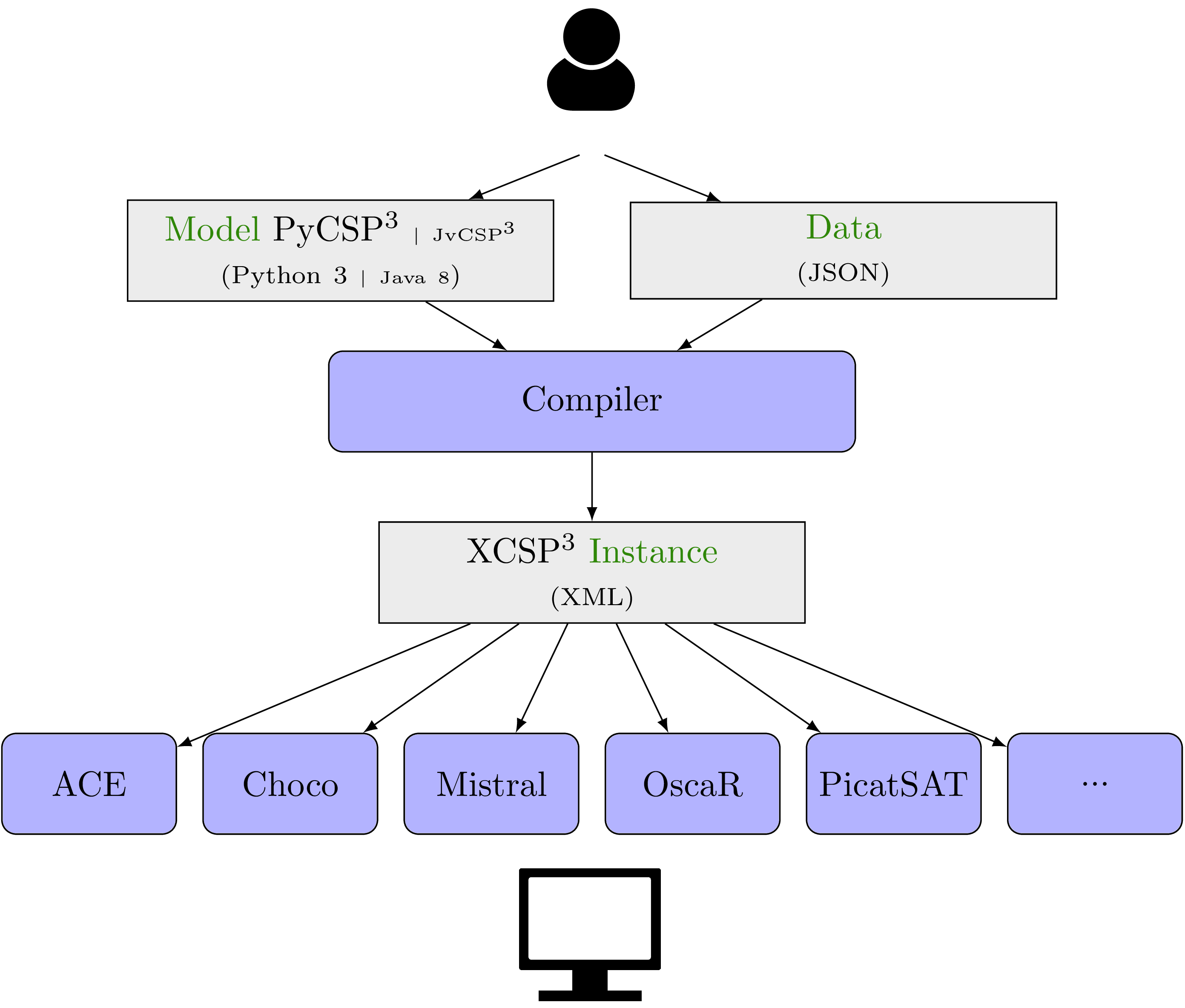# PyCSP3

PyCSP3 is a Python library that allows us to write models of combinatorial constrained problems in a declarative manner. Currently, with PyCSP3, you can write models of constraint satisfaction and optimization problems. More specifically, you can build models of:

• CSP (Constraint Satisfaction Problem)
• COP (Constraint Optimization Problem)

You can find two examples of PyCSP3 models below

#### AllInterval problem


from pycsp3 import *

n = data

# x[i] is the ith note of the series
x = VarArray(size=n, dom=range(n))

satisfy(
# notes must occur once, and so form a permutation
AllDifferent(x),

# intervals between neighbouring notes must form a permutation
AllDifferent(abs(x[i] - x[i + 1]) for i in range(n - 1)),

# tag(symmetry-breaking)
x < x[n - 1]
)



#### Golomb Ruler problem


from pycsp3 import *

n = data

# x[i] is the position of the ith tick
x = VarArray(size=n, dom=range(n * n))

satisfy(
# all distances are different
AllDifferent(abs(x[i] - x[j]) for i, j in combinations(range(n), 2)),

# tag(symmetry-breaking)
[x == 0, Increasing(x, strict=True)]
)

minimize(
# minimizing the position of the rightmost tick
x[-1]
)


A shown in the figure below, the user who wishes to solve a combinatorial constrained problem has to:

• write a model using the Python library PyCSP3 (i.e., write a Python file), or the Java modeling API JvCSP3 (i.e., write a Java file)
• provide a data file (in JSON format) for a specific problem instance to be solved
• compile both files (model and data) so as to generate an XCSP3 instance (file)
• solve the XCSP3 file (problem instance under format XCSP3) by using a constraint solver as, e.g., AbsCon, Choco, OscaR or PicatSAT